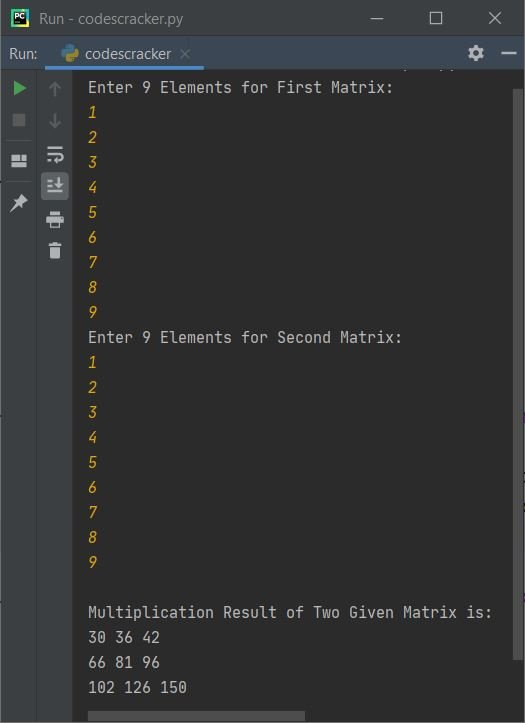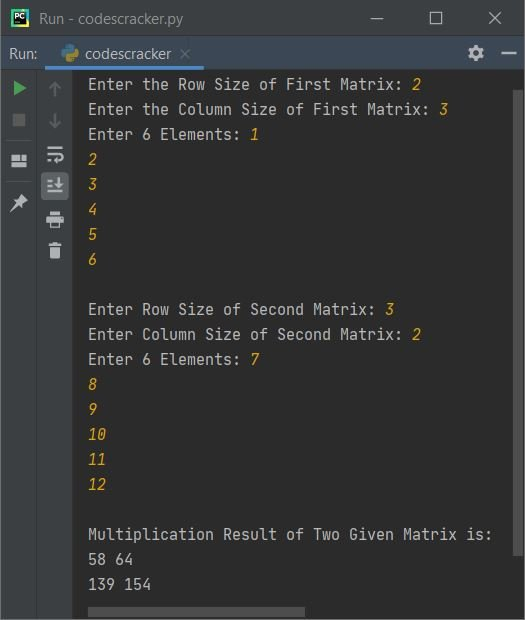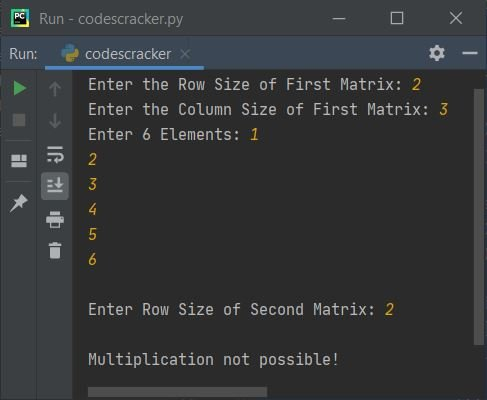# Python Program to Multiply Two Matrices

• Multiply Two 3*3 Matrices entered by user
• Multiply Two Matrices of Given Size and Elements, by user at run-time

Before creating these programs, if you're not aware about steps used to define matrix multiplication, then refer to Matrix Multiplication Steps and Example to get every required thing about the topic. Now let's move on and create a Python program to implement matrix multiplication.

## Multiply Two 3*3 Matrices entered by User

To perform matrix multiplication or to multiply two matrices in Python, you have to ask from user to enter 9-9 elements for both matrices one by one. Then perform the operation of matrix multiplication and print the result like shown in the program given below:

```matOne = []
print("Enter 9 Elements for First Matrix: ")
for i in range(3):
matOne.append([])
for j in range(3):
num = int(input())
matOne[i].append(num)

matTwo = []
print("Enter 9 Elements for Second Matrix: ")
for i in range(3):
matTwo.append([])
for j in range(3):
num = int(input())
matTwo[i].append(num)

matThree = []
for i in range(3):
matThree.append([])
for j in range(3):
sum = 0
for k in range(3):
sum = sum + (matOne[i][k] * matTwo[k][j])
matThree[i].append(sum)

print("\nMultiplication Result of Two Given Matrix is:")
for i in range(3):
for j in range(3):
print(matThree[i][j], end=" ")
print()```

Here is its sample run:Now supply the input say 1, 2, 3, 4, 5, 6, 7, 8, 9 as nine elements for first and then same nine elements for second matrix to perform the multiplication and print the multiplication result like shown in this snapshot given below:From above program, the following statement:

`matOne = []`

defines an empty list named matOne. And the following statement:

`matOne.append([])`

appends an empty list as an element. This statement is used to store all 9 elements to list matOne in 3-3 pairs. That is, following statements:

```matOne = []
matOne.append([])```

indicates to:

`matOne[[]]`

Therefore after entering all nine (say 1, 2, 3, 4, 5, 6, 7, 8, 9) elements for first matrix. Elements gets stored in matOne in this way:

`matOne[[1, 2, 3], [4, 5, 6], [7, 8, 9]]`

Rest of the things from above program are understandable. If you're finding trouble while reading the code, that is applied for actual multiplication, then refer to matrix multiplication article to get idea about steps used there.

## Multiply Two Matrices of Given Size

This program allows user to define the size of both matrices along with its elements. The question is, write a Python program to perform matrix multiplication of given size and elements by user. Here is its answer:

```print("Enter the Row and Column Size of First Matrix: ", end="")
rOne = int(input())
cOne = int(input())
print("Enter " +str(rOne * cOne)+ " Elements: ", end="")
mOne = []
for i in range(rOne):
mOne.append([])
for j in range(cOne):
num = int(input())
mOne[i].append(num)

print("\nEnter Row and Column Size of Second Matrix: ", end="")
rTwo = int(input())
if cOne != rTwo:
print("\nMultiplication Not Possible!")
exit()
else:
cTwo = int(input())
print("Enter " +str(rTwo * cTwo)+ " Elements: ", end="")
mTwo = []
for i in range(rTwo):
mTwo.append([])
for j in range(cTwo):
num = int(input())
mTwo[i].append(num)

# now multiplying two matrices
mThree = []
for i in range(rOne):
mThree.append([])
for j in range(cTwo):
sum = 0
for k in range(cOne):
sum = sum + (mOne[i][k] * mTwo[k][j])
mThree[i].append(sum)

# now printing the multiplication result
print("\nMultiplication Result of Two Given Matrix is:")
for i in range(rOne):
for j in range(cTwo):
print(mThree[i][j], end=" ")
print()```

What if user enters an invalid input like #, a, or \ etc. as row size, column size, or even as element ? Therefore let's modify this program to handle with invalid inputs too.

#### Modified Version of Previous Program

This is the modified version of previous program. This program is created to print message regarding to every part of invalid inputs using try-except. That is, the first statement of try gets executed, if that raised an error, then program flow immediately goes to its except counterpart and execute the statement inside it.

```print("Enter the Row Size of First Matrix: ", end="")
try:
rOne = int(input())
print("Enter the Column Size of First Matrix: ", end="")
try:
cOne = int(input())
print("Enter " +str(rOne * cOne)+ " Elements: ", end="")
mOne = []
for i in range(rOne):
mOne.append([])
for j in range(cOne):
try:
num = int(input())
mOne[i].append(num)
except ValueError:
print("\nInvalid Input!")
exit()
print("\nEnter Row Size of Second Matrix: ", end="")
try:
rTwo = int(input())
if cOne == rTwo:
print("Enter Column Size of Second Matrix: ", end="")
try:
cTwo = int(input())
print("Enter " +str(rTwo * cTwo)+ " Elements: ", end="")
mTwo = []
for i in range(rTwo):
mTwo.append([])
for j in range(cTwo):
try:
num = int(input())
mTwo[i].append(num)
except ValueError:
print("\nInvalid Input!")
exit()

# now multiplying two matrices
mThree = []
for i in range(rOne):
mThree.append([])
for j in range(cTwo):
sum = 0
for k in range(cOne):
sum = sum + (mOne[i][k] * mTwo[k][j])
mThree[i].append(sum)

# now printing the multiplication result
print("\nMultiplication Result of Two Given Matrix is:")
for i in range(rOne):
for j in range(cTwo):
print(mThree[i][j], end=" ")
print()
except ValueError:
print("\nInvalid Column Size!")
else:
print("\nMultiplication not possible!")
except ValueError:
print("\nInvalid Row Size!")
except ValueError:
print("\nInvalid Column Size!")
except ValueError:
print("\nInvalid Row Size!")```

Here is its sample run with user inputs 2 as row size and 3 as column size of first matrix, then 1, 2, 3, 4, 5, 6 as six elements of first matrix. Similarly 3 as row size and 2 as column size of second matrix, then 7, 8, 9, 10, 11, 12 as six elements for second matrix:Here is another sample run:#### Same Program in Other Languages

Python Online Test

« Previous Program Next Program »